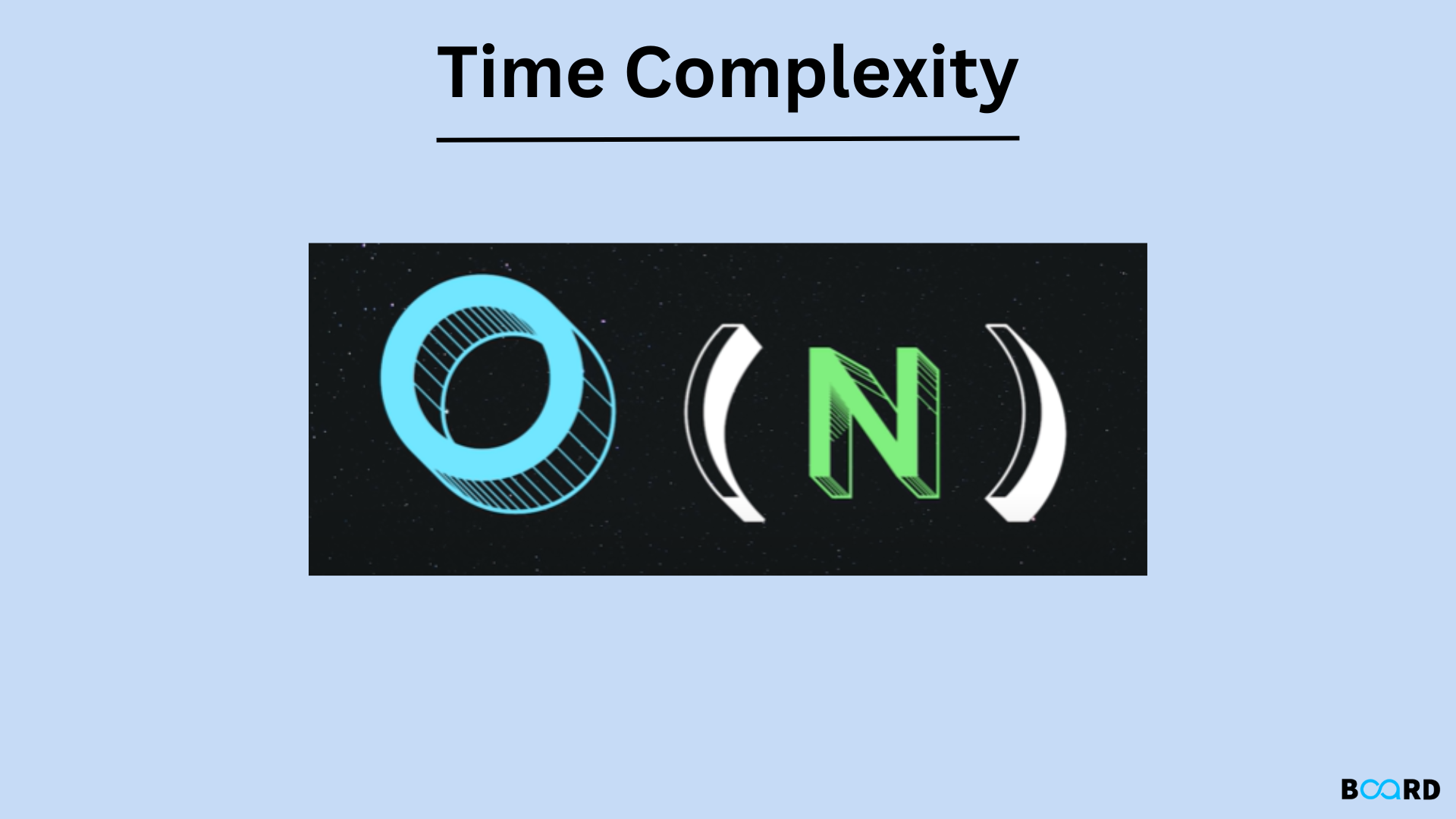# Time Complexity: Explanation## Definition

The analysis of an algorithm's effectiveness is called time complexity. It reveals how long an algorithm needs to process a particular input. We can determine the relative merits of various algorithms using asymptotic notation.

Let's now examine the definition of "order of" in mathematics. There are primarily three types of asymptotic notations that are frequently used.

• Big oh symbol ( O )
• The big omega symbol ( Ω )
• Big theta one ( θ ) - The common one.

## Big oh notation (0)

Simply put, big O in our industry refers to "order of," although this differs significantly from the mathematical concept of the big O. In mathematics, "big O" represents all the intricacies that our program must navigate. However, in business, we only ask the bare minimum of them. Thus, this was a slight variation.

If O(1) and O(n) were plotted on a graph, they would resemble the following

• An asymptotic upper bound is expressed in terms of big O notation.
• If an algorithm's running time is expressed mathematically as f(n), then f(n) is O(g(n)) if and only if there are positive constants c and n° that allow for the following:
 0 ≤ f(n) ≤ c g(n)        for all n ≥ n°
• Here, n is the size of the input, and g(n) denotes any complexity function, such as n, n2, etc.
• If a function is O(n), it is also O(n2) (used to establish an upper bound on a function) because it fulfills the aforementioned equation.

## Big Omega Notation (Ω)

• The asymptotic lower bound in notation is equivalent to the asymptotic higher bound in O notation.
• Let f(n) represent the algorithm's running time; f(n) is said to be (g(n)) if and only if c and n° are positive constants such that
 0 ≤ c g(n) ≤ f(n)        for all n ≥ n°
• It is applied to provide a function's lower bound.
• A function that fulfills the aforementioned equation and is (n2) is also (n) by default.

## Big theta notation (Θ)

• It is applied to provide a function's lower bound.
• A function that fulfills the aforementioned equation and is (n2) is also (n) by default.

### Mathematically

 0  ≤ c2 g(n)  ≤  f(n) ≤ c1 g(n)      ∀    n ≥ no

The equation simply states that positive constants c1 and c2 must exist in order for f(n) to be sandwiched between c2 g(n) and c1 g. (n).

Following are some typical runtimes that you will encounter in your programming career, listed in increasing order.

So the asymptotic notations were the main focus of this. These will be a common occurrence in the future.